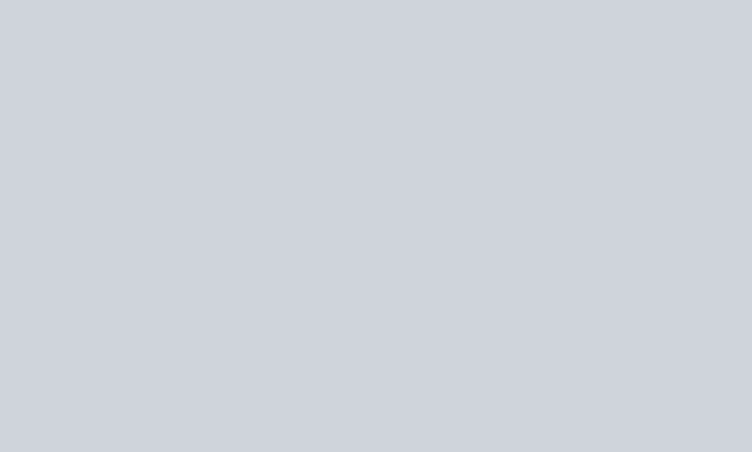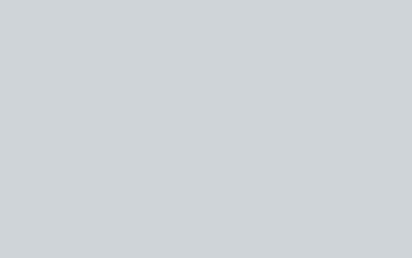# Maths Questions (English)

Quantitative Aptitude – Maths Questions for SSC CHSL and CUET in (English) to test Basic Arithmetic Skill. Online Practice Set : Questions and Answers from previous year exam question as per latest syllabus and exam pattern.

### Set 1 :Maths Questions

Q.1: When (224 – 1) is divided by 7, the remainder is :
(A) 2
(B) 0
(C) 4
(D) 1

Ans : (B) 0

Q.2: If a + b + c = 7 and a3 + b3 + c3 – 3abc = 301, then ab + bc + ca = ?
(A) -4
(B) -2
(C) 3
(D) 2

Ans : (D) 2

Q.3: Study the given graph and answer the question that follows.By what percentage is the total exports in 2014 and 2016 less than the total imports in the same two years ?

(A) 12.5
(B) 12.9
(C) 14.3
(D) 11.25

Ans : (A) 12.5

Q.4: In a circle with centre 0, chord AB and diameter CD intersect each other at point E, inside the circle, If$\angle\text{AOD} = 42^o\text{and}\angle\text{BOC} = 104^o$ , then what is the measure (in degrees) of$\angle\text{AED}$ ?
(A) 73
(B) 62
(C) 58
(D) 84

Ans : (A) 73

Q.5: If$x^2-3\sqrt2x+1=0$, then what is the value of$x^3+(\frac{1}{x^3})$?
(A)$30\sqrt6$
(B)$45\sqrt2$
(C)$15\sqrt6$
(D)$30\sqrt2$

Ans : (B)$45\sqrt2$

Q.6: If x2 + 1 – 2x = 0, x > 0, then x2(x2 – 2)= ______.
(A) 1
(B)$\sqrt2$
(C) -1
(D) 0

Ans : (C) -1

Q.7: Chords AB and CD of a circle intersect externally at P. If CD=11.6 cm, PD=6.4 cm and PB=7.2 cm, then AB (in cm) is equal to:
(A) 12
(B) 16
(C) 8.8
(D) 4.8

Ans : (C) 8.8

Q.8: If$cos^2\theta=3(1-sin\theta), 0^o <\theta <90^o$, then what is the value of$(tan2\theta+cosec3\theta-sec2\theta)$ ?
(A)$\sqrt3+1$
(B)$\sqrt3-1$
(C)$\frac{1+\sqrt3}{\sqrt3}$
(D)$\frac{1-\sqrt3}{\sqrt3}$

Ans : (B)$\sqrt3-1$

Q.9: the value of$8\div2 of 3-[56\div4 of {4\times3-(9 - 11)\div(2\div4 of 4)}]$ is:
(A)$\frac{11}{6}$
(B)$\frac56$
(C)$\frac43$
(D)$\frac12$

Ans : (B)$\frac56$

Q.10: The perimeters of two similar triangles ABC and PQR are 156cm and 46.8 cm, respectively. If BC = 19.5 cm and QR = x cm, then the value of x is:
(A) 5.85 cm
(B) 6.75 cm
(C) 3.76 cm
(D) 4.29 cm

Ans : (A) 5.85 cm

Q.11: A can complete a certain work in 30 days. He started the work. After 4 days, B joined him and the whole work was completed in 10 days from the beginning, B alone can complete one-third of the same work in:
(A) 6 days
(B) 3 days
(C) 4 days
(D) 2 days

Ans : (B) 3 days

Q.12: If the different between the compound interest compounded annually and the simple interest on a certain sum of money for three years at 10% per annum is ₹279, then the sum (in ₹) is:
(A) 10,000
(B) 8,000
(C) 7,500
(D) 9,000

Ans : (D) 9,000

Q.13: In$\triangle\text{ABC},\angle A = 90^o$, AB = 20 cm and BC = 29 cm. What is the value of (sinB – cotC) ?
(A)$-\frac{9}{29}$
(B)$-\frac{189}{580}$
(C)$\frac{189}{580}$
(D)$\frac{9}{29}$

Ans : (B)$-\frac{189}{580}$

Q.14: In a constituency, 55% of the total number of voters are males and the rest are females. If 40% of the males are illiterate and 40% of the females are literate, then by what percentage is the number of illiterate females more than that of the illiterate males (correct to one decimal place)?
(A) 22.7%
(B) 16.4%
(C) 21.5%
(D) 20.8%

Ans : (A) 22.7%

Q.15: By selling 3 dozen oranges for ₹405, a trader loses 25%. How many oranges should he sell for ₹288 if he needs to earn a profit of 20% in the transaction?
(A) 18
(B) 15
(C) 16
(D) 12

Ans : (C) 16

Q.16: X and Y start at the same time to ride from place A to place B, Which is 80 km away from A. X travels 4 km per hour slower than Y. Y reaches place B and at once turns back meeting X, 16 km from place B. Y’s speed (in km/h) is:
(A) 9
(B) 15
(C) 8
(D) 12

Ans : (D) 12

Q.17: There are three numbers. If the ratio of the first to the second number is 2 : 7 and that of the second to the third number is 5 : 8, then the ratio of the first to the third number is:
(A) 7 : 8
(B) 5 : 28
(C) 1 : 4
(D) 5 : 7

Ans : (B) 5 : 28

Q.18: Study the given graph and answer the question that follows.What is the ratio of the total production of paper by company A in 2016 and 2019 to the total production of paper by company B in 2014 and 2017 ?

(A) 9 : 7
(B) 3 : 2
(C) 5 : 4
(D) 8 : 5

Ans : (A) 9 : 7

Q.19: BD and CE are the medians of$\triangle\text{ABC}$, right angled at A. If CE$=\frac{5\sqrt{13}}{2}$ cm, BC=10 cm, then the length of BD is:
(A)$3\sqrt7$ cm
(B)$3\sqrt5$ cm
(C)$\sqrt{13}$ cm
(D)$\frac52\sqrt7$ cm

Ans : (D)$\frac52\sqrt7$ cm

Q.20: The following histogram represents the daily earnings of 50 medical stores in a locality.The difference between the percentage of medical stores whose daily earnings are less than ₹1,000 and the percentage of medical stores whose daily earnings are ₹1,200 and above is:

(A) 24%
(B) 16%
(C) 15%
(D) 12%

Ans : (B) 16%

Q.21: The marked price of an article is ₹480. A person buys it at two successive discounts of 15% and 10%. He sells it at a profit of 25%. What is his profit (in ₹) ?
(A) 95.40
(B) 90.60
(C) 91.80
(D) 93.20

Ans : (C) 91.80

Q.22: The average of 19 number is 48. The average of the first 7 numbers is 50.6 and that of the last 13 numbers is 47.6. If the 7th number is excluded, then what is the average of the remaining numbers (correct to one decimal place)?
(A) 42.4
(B) 49.5
(C) 39.6
(D) 47.3

Ans : (D) 47.3

Q.23: Study the following bar graph and answer the question given below.
The bar graph represents the total number of applications received for B.SC, Mathematics by two different colleges A and B in 5 different years.Find the difference between the average number of applications received by college A from 2014 to 2018 and the average number of applications received by college B from 2014 to 2018 ?

(A) 5
(B) 4
(C) 6
(D) 8

Ans : (B) 4

Q.24: As observed from the top of a lighthouse. 42 m high above sea-level, the angle of depression of a ship sailing directly towards it changes from 30o to 45o. The distance travelled by the ship during the period of observation is:
(A)$42(1-\sqrt3)$
(B)$42(\sqrt3+1)$
(C)$42(\sqrt3-1)$
(D) 42

Ans : (C)$42(\sqrt3-1)$

Q.25: What is the cost (in ₹) of levelling a triangular park with sides 60 m, 112m and 164 m at the rate of ₹10.50 per m2 ?
(A) 21,063
(B) 20,160
(C) 21,168
(D) 21,000